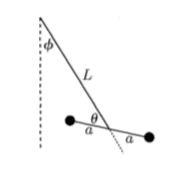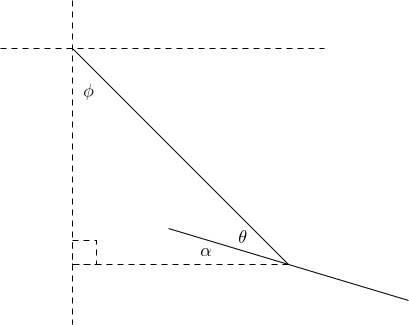# Angular momentum of two particles connected by a rigid bar

Jenny Physics
Homework Statement:
Find the angular momentum of the system of two particles in the figure connected by a (massless) rigid bar.
Relevant Equations:
$$L=r_{left}\times mv_{left}+r_{right}\times mv_{right}$$Lets do it for the left (the right will be similar): ##r_{left}=[(L-a\sin\theta)\sin\phi,(L+a\cos\theta)\cos\phi]## so ##v_{left}=[-a\dot{\theta}\cos\theta\sin\phi+(L-a\sin\theta)\dot{\phi}\cos\phi,-a\dot{\theta}\sin\theta\cos\phi-(L+a\cos\theta)\dot{\phi}\sin\phi]##. Is this right?

Last edited:

Homework Helper
Gold Member
2022 Award
How is this system moving?

Jenny Physics
How is this system moving?
It is a pendulum swinging back and forth and so both ##\phi,\theta## change with time.

Staff Emeritus
Homework Helper
Gold Member
Are you trying to find just the angular momentum or go via the Lagrangian of the system?

Jenny Physics
Are you trying to find just the angular momentum or go via the Lagrangian of the system?
Just trying to find the angular momentum using the definition.

Homework Helper
Gold Member
2022 Award
I assume this is all in one plane.
You have omitted a from your expressions, and anyway your expressions for the r coordinates are wrong. Draw a diagram showing where ##(L-a\sin(\theta))\sin(\phi)## is.

But I think it will be much easier treating both particles together and using the parallel axis theorem.

•Jenny Physics and PeroK
Homework Helper
Gold Member
2022 Award
Homework Statement:: Find the angular momentum of the system of two particles in the figure connected by a (massless) rigid bar.
Homework Equations:: $$L=r_{left}\times mv_{left}+r_{right}\times mv_{right}$$

View attachment 254771
Lets do it for the left (the right will be similar): ##r_{left}=[(L-a\sin\theta)\sin\phi,(L+a\cos\theta)\cos\phi]## so ##v_{left}=[-a\dot{\theta}\cos\theta\sin\phi+(L-a\sin\theta)\dot{\phi}\cos\phi,-a\dot{\theta}\sin\theta\cos\phi-(L+a\cos\theta)\dot{\phi}\sin\phi]##. Is this right?

Try ##\theta = 0## in your equation for ##r_{left}##. Something is not right there.

•Jenny Physics
Staff Emeritus
Homework Helper
Gold Member
Also, no, the expressions are not correct. You cannot have factors such as ##L-\cos\theta## as the physical dimensions of the terms are different.

Jenny Physics
It should then be ##r_{left}=[(L-a\cos\theta)\sin\phi,(L-a\cos\theta)\cos\phi]##? That will not work when ##\theta=\pi/2##

Homework Helper
Gold Member
2022 Award
It should then be ##r_{left}=[(L-a\cos\theta)\sin\phi,(L-a\cos\theta)\cos\phi]##? That will not work when ##\theta=\pi/2##
Wild guesses won't get you there.
Draw a horizontal line through the top pivot (O) and vertical lines up to that from the left mass and the lower pivot, meeting it at A, B. Can you figure out the lengths of OB, AB?

•Jenny Physics
Staff Emeritus
Homework Helper
Gold Member
Wild guesses won't get you there.
Draw a horizontal line through the top pivot (O) and vertical lines up to that from the left mass and the lower pivot, meeting it at A, B. Can you figure out the lengths of OB, AB?
I still think the parallel axis theorem is a better way forward, as you mentioned in #6.

•Jenny Physics
Jenny Physics
Wild guesses won't get you there.
Draw a horizontal line through the top pivot (O) and vertical lines up to that from the left mass and the lower pivot, meeting it at A, B. Can you figure out the lengths of OB, AB?
The length of ##OB=L\sin\phi##. The length of AB requires some trigonometry involving ##\theta## which is not obvious to me.

Staff Emeritus
Homework Helper
Can you figure out the angle ##\alpha## in this diagram in terms of ##\phi## and ##\theta##?•Jenny Physics
Jenny Physics
Can you figure out the angle ##\alpha## in this diagram in terms of ##\phi## and ##\theta##?

View attachment 254779
##\phi+\theta+\alpha=\pi/2## that would mean that ##\alpha=\pi/2-\phi-\theta## and hence ##AB=a\cos\alpha=a\sin(\phi+\theta)##?

•